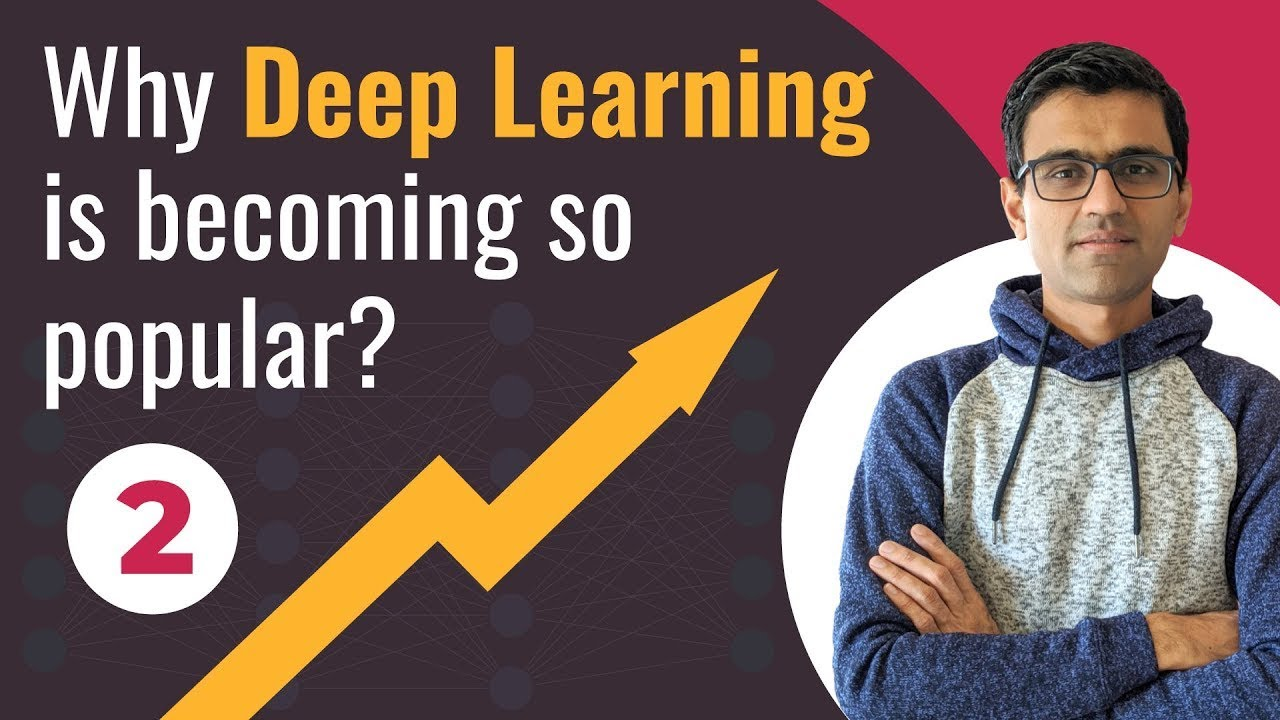# Why Deep Learning is Becoming so Popular? | Deep Learning Tutorial (TensorFlow 2.0, Keras & Python)This video explains four reasons why deep learning has become so popular in past few years. In this deep learning tutorial python, I will cover following things in this video: Introduction; Data growth; Hardware advancements; Python and opensource ecosystem; Cloud and AI boom

This video explains four reasons why deep learning has become so popular in past few years. In this deep learning tutorial python, I will cover following things in this video,

• 00:00 Introduction
• 00:24 Data growth
• 02:40 Python and opensource ecosystem
• 04:00 Cloud and AI boom

## Pytorch vs Tensorflow vs Keras | Deep Learning Tutorial (Tensorflow, Keras & Python)

We will go over what is the difference between pytorch, tensorflow and keras in this video. Pytorch and Tensorflow are two most popular deep learning frameworks. Pytorch is by facebook and Tensorflow is by Google. Keras is not a full fledge deep learning framework, it is just a wrapper around Tensorflow that provides some convenient APIs.

## Keras with TensorFlow Course - Python Deep Learning and Neural Networks

Keras with TensorFlow Course - Python Deep Learning and Neural Networks for Beginners Tutorial: How to use Keras, a neural network API written in Python and integrated with TensorFlow. We will learn how to prepare and process data for artificial neural networks, build and train artificial neural networks from scratch, build and train convolutional neural networks (CNNs), implement fine-tuning and transfer learning, and more!

## Transfer Learning | Deep Learning Tutorial (TensorFlow, Keras & Python)

In this video we will go over some theory behind transfer learning and then use google's mobile net v2 pre trained model to train our flowers dataset 📺 Transfer learning is a very important concept in the field of computer vision and natural language processing. Using transfer learning you can use pre trained model and customize it for your needs. This saves computation time and money. It has been a revolutionary break through in the field of deep learning and nowadays you see it being used widely in the industry.

## Introduction | Deep Learning Tutorial (TensorFlow, Keras & Python)

With this video, I am beginning a new deep learning tutorial series for total beginners. In this deep learning tutorial python, I will cover following things in this series: 1. Explain neural network concepts in most easiest way 2. Go over math if needed, otherwise keep the tutorials simple and easy 3. Provide exercises that you can practice on 4. Use python, keras and tensorflow mainly. I might cover pytorch as well 5. Cover convolutional neural network (CNN) for image and video processing 6. Cover recurrent neural network (RNN) for sequential analysis and natural language processing (NLP)

## Implement Neural Network In Python | Deep Learning Tutorial (TensorFlow 2.0, Keras & Python)

In this video we will implement a simple neural network with single neuron from scratch in python. This is also an implementation of a logistic regression in python from scratch. You know that logistic regression can be thought of as a simple neural network. The pre requisite for this tutorial is the previous tutorial on gradient descent (link below). We will be using gradient descent python funciton written in previous video to implement our own custom neural network class.